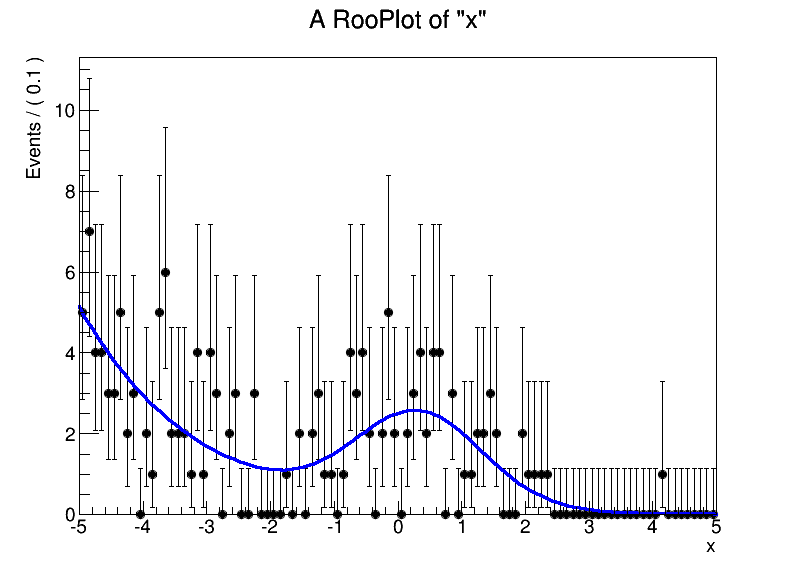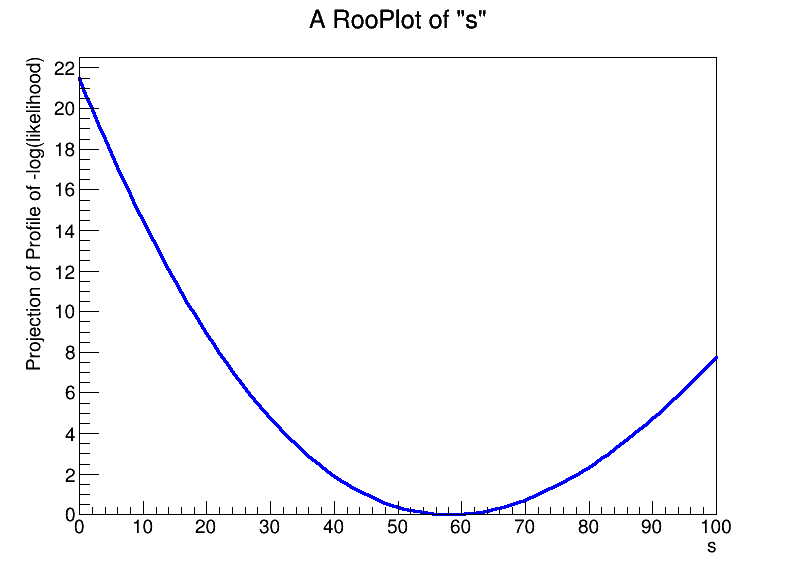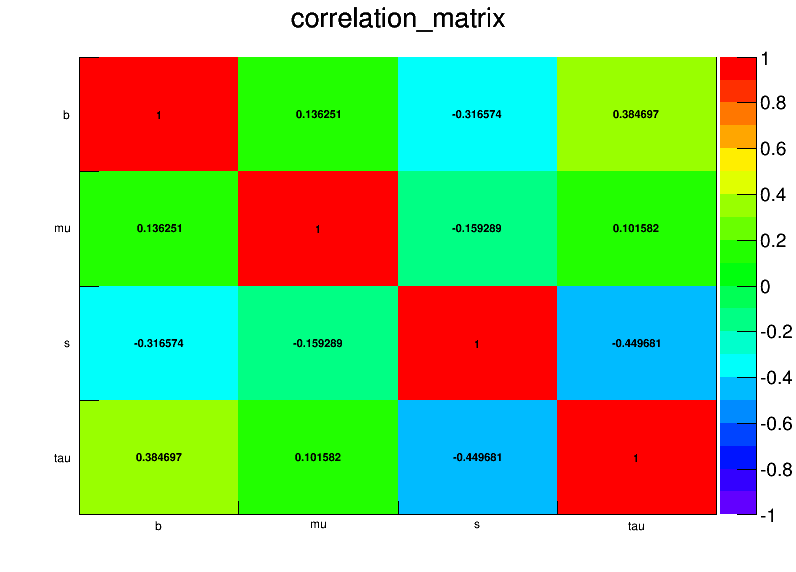Particle physicisits primarily use ROOT for the data analysis framework. Part of that framework is a package called RooFit statistical modeling and fitting package. I have contributed to this package and added a layer on top called RooStats that provides with statistical inference in both frequentist and Bayesian paradigms based on statistical models made with RooFit. These are the tools that were used to claim the discover the Higgs boson, and those statistical models get pretty complicated.

I created a little IPython notebook to demonstrate a simple example of RooFit's ability to create a statistical model, generate some simulated data, fit that data, create the profile likelihood, and provide a covariance matrix from the likelihood fit.  Enjoy!

For convenience, here it is embeded

# A basic example of ROOT's RooFit statistical modeling language in iPython notebook¶

License: BSD (C) 2014, Kyle Cranmer. Feel free to use, distribute, and modify with the above attribution.

Particle physicisits primarily use ROOT for the data analysis framework. Part of that framework is a package called RooFit statistical modeling and fitting package. I have contributed to this package and added a layer on top called RooStats that provides with statistical inference in both frequentist and Bayesian paradigms based on statistical models made with RooFit. These are the tools that were used to claim the discover the Higgs boson, and those statistical models get pretty complicated.

Here I demonstrate a simple example of RooFit's ability to create a statistical model, generate some simulated data, fit that data, create the profile likelihood, and provide a covariance matrix from the likelihood fit.

ROOT is a C++ library, but it has python bindings known as PyROOT.

In :
import ROOT
import rootnotes #for plotting with iPython
c1 = rootnotes.default_canvas()


Here we create a "workspace" object that provides a factory with a convenient syntax for statistical models and variables. The workspace also provides an I/O mechanism to read/write statistical models and data to/from files.

In this example, we create a mixture model of a falling exponential distribution and a Gaussian for a variable x.
This is really a marked Poisson process, because in addition to the pdf on x, we also encode that we expect s=50 events from the Gaussian and b=100 events from the falling exponential.

In :
w = ROOT.RooWorkspace()
w.factory('Gaussian::g(x[-5,5],mu[-3,3],sigma)')
w.factory('Exponential::e(x,tau[-.5,-3,0])')
w.factory('SUM::model(s[50,0,100]*g,b[100,0,1000]*e)')
w.Print() #this isn't displaying in iPython

TClass::TClass:0: RuntimeWarning: no dictionary for class stack > is available


Now that we have made the mdoel, we can generate some fake data, make a plot of the data, fit the model to that data, and overlay the fitted model in the plot

In :
x = w.var('x')
pdf = w.pdf('model')
frame = x.frame()
data = pdf.generate(ROOT.RooArgSet(x))
data.plotOn(frame)
fitResult = pdf.fitTo(data,ROOT.RooFit.Save())
pdf.plotOn(frame)
frame.Draw()
c1

Out:We can check the value of the parameters after the fit

In :
mu = w.var('mu')
print 'best fit value of mean for Gaussian is', mu.getVal(), '±', mu.getError()

best fit value of mean for Gaussian is 0.324840840875 ± 0.158716435359


And make a plot of the log(profile likelihood Ratio) as a function of the signal rate

In :
s=w.var('s')
sframe=s.frame()
lnL = pdf.createNLL(data)
lnProfileL = lnL.createProfile(ROOT.RooArgSet(s))
lnProfileL.plotOn(sframe)
sframe.Draw()
c1

Out:And finally, make a plot that encodes the correlation matrix between the four free parameters in the fit.

In :
corrMatrixHist = fitResult.correlationHist()
corrMatrixHist.SetStats(False)
corrMatrixHist.Draw('colz text')
c1

Out:Well, I hope that's interesting. It's just a taste of what's possible with RooFit and RooStats.

This post was created entirely in IPython notebook. Download the raw notebook here, or see a static view on nbviewer.